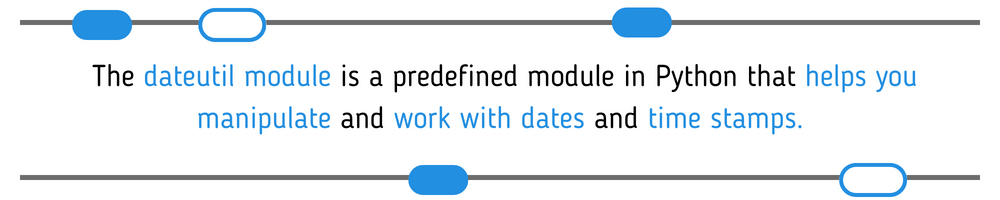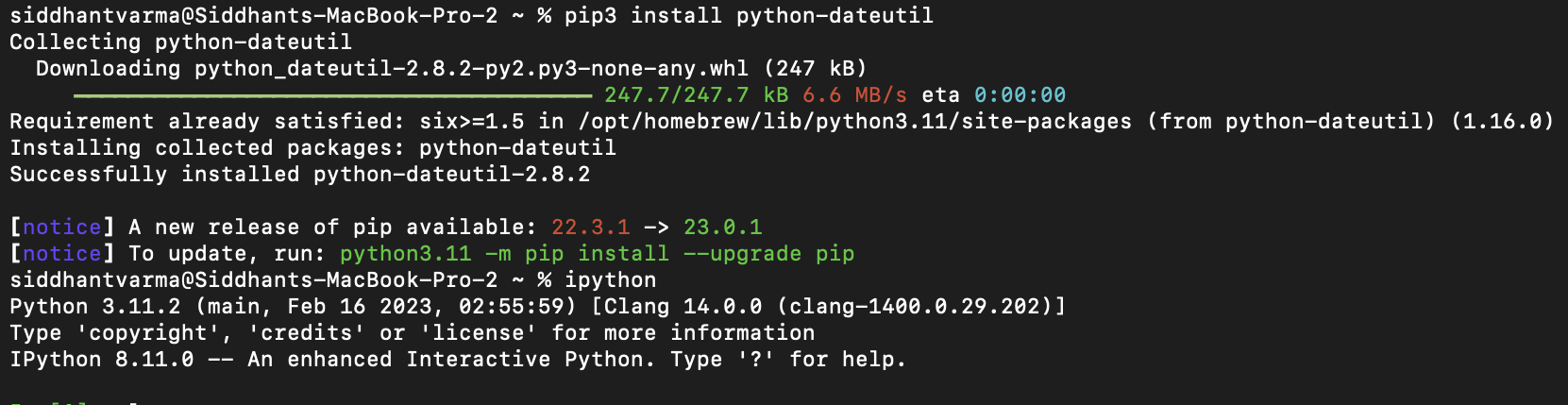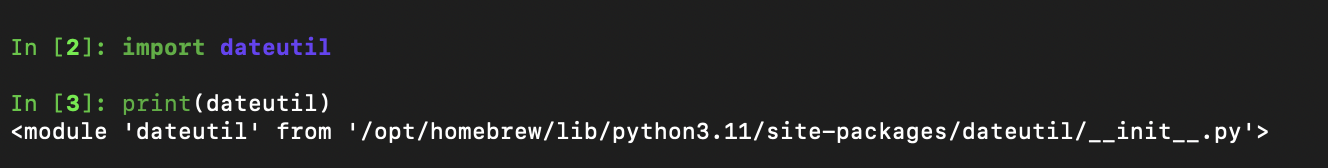# A Guide to Working with the Dateutil Module in Python

This post was written by Siddhant Varma. Scroll down to view the author’s profile.

Python is a highly versatile language. From software engineering to machine learning and data analysis, it’s everywhere. As a multipurpose scripting and programming language, it’s often utilized for manipulating and working with data. So, when you’re working with Python, whether you’re analyzing data or writing scripts, you’re likely to encounter dates and time stamps.

Dealing with dates can be challenging if you attempt to create your own logic for manipulating them from scratch. To simplify this process, Python offers a dateutil module. In this post, we’ll teach you about the dateutil module, what it is, and how it operates. Then we’ll show you some common examples of frequently used date functions that you can implement using the dateutil module.## What is the dateutil module in Python?

The dateutil module is a predefined module in Python that helps you manipulate and work with dates and time stamps. You can use it for a number of purposes when working with dates, such as adding dates, parsing dates in different formats, subtracting dates, calculating the difference between two dates, etc. We’ll explore some common examples later in the post.

The dateutil module is an extension of the datetime module.

## Is dateutil included in Python 3?

The dateutil module is not a part of Python 3’s standard library, but that doesn’t mean you can’t use it. You can easily install it using pip. Once you do that, you can import the dateutil module into any of your Python scripts using the standard import statement the way you normally use other Python modules.

We’ll show you how you can use the dateutil module with Python 3 in the section below.

## How does it work?

First, we’ll show you how dateutil works under the hood since it’s an abstraction of the datetime module. The dateutil module was built on top of the datetime module by adding some functions and classes to the existing functions and classes. These functions and classes make it easier to work with dates and times in a variety of ways. For example, dateutil includes functions for parsing dates and times from strings in a wide variety of formats.

Now we’ll show you how to install the dateutil module in Python 3.

For this tutorial, I’m using pip3 and Python 3, but you can follow the equivalent steps and code for other versions of Python. First, install the dateutil module using pip3 by running the following command:

``pip3 install python-dateutil``

Running that command should install the dateutil module for you.Now, in order to verify the installation and check that the dateutil module is correctly installed, you can try to import the module. I’m using ipython to run these Python commands directly in the terminal, but you can also create a .py file and run it.

``````import dateutil

print(dateutil)``````

If dateutil is somehow not correctly installed, the above program will throw an error. Otherwise, it should give you the path reference where the dateutil module exists in your system.## Using the dateutil module

Now that you’ve installed the module, let’s look at how you can use it with examples.

### Parsing dates and times

The dateutil module provides a parse function that you can use to parse dates into desired string formats. First, you’ll need to import the parse function:

``from dateutil.parser import parse``

Then you can use the parse function to return a parsed version of the date string. Take a look at the following code:

``````today = "2022-03-05"

parsed_today = parse(today)
print(parsed_today)``````

When you print the value of parsed_today, you should get the date string parsed in ISO format as shown below:

``2022-03-05 00:00:00``

Here’s how you can use the parse function to parse a date string in a relative format:

``````date_relative = "next sunday  at 4pm"

parsed_date = parse(date_relative, fuzzy=True)
print(parsed_date)``````

In the code above, we pass the fuzzy parameter as true to the parse function, which tells the parse function to generate the formatted date based on the date string passed.

### Calculating the difference between two dates

Now we’ll show you how you can compute the difference between two dates using the dateutil module. This is a common operation to find the relative time elapsed of a date from a certain date.

First, import the relativedelta function from the dateutil module:

``from dateutil.relativedelta import relativedelta``

The relativedelta function will be used to calculate the difference between two date strings. Have a look at the following code:

``````date1 = parse("2022-03-05")

date2 = parse("2022-03-10")
diff = relativedelta(date2, date1)
print(diff)``````

Let’s see what the output is:

``relativedelta(days=+5)``

If you look closely, the diff variable reveals the difference between the two dates, i.e., five days. But let’s only print the difference in days between these two dates:

``print(diff.days)``

The diff itself is an object, and the days property gives you the difference in the number of days. In a similar way, you can find the difference between two dates in months and years by accessing the months and years property on the difference variable.

### Adding and subtracting time from a date

Another common date manipulation that you might need is adding or subtracting time from a given date. We’ll now show you how you can add a specific number of days to the current date:

``````from datetime import date, timedelta
current_date = date(2022, 3, 5)
delta = timedelta(days=7)
new_date = current_date + delta
print(new_date)``````

That should give you a date seven days in the future from today:

``2022-03-12``

Awesome! Similarly, you can subtract a specific number of days from a given date:

``````from datetime import date, timedelta
current_date = date(2022, 3, 5)
delta = timedelta(days=7)
new_date = current_date - delta
print(new_date)``````

And that should give you a date seven days in the past:

``2022-02-26``

Great!

## Practical application of the dateutil module

By now you’ve seen enough examples to understand how the dateutil module works. But what’s the practical use case or real-life scenario where you’d want to use it? You can use the dateutil module to work with a time series database.

A time series database is a special kind of database that’s optimized for storing and querying time series data. Unlike regular data that’s only written into the database once when needed, time series data is recorded and stored in the database over time. Some common examples of time series data include data that you need to measure from an Internet of Things sensor, the changing prices of a stock over a period of a few days, weather data that’s updated daily, and so on. Time series databases are designed to handle large volumes of data that are recorded at regular intervals.

Curious to learn more and get your hands dirty with a time series database? Check out Influx DB. It allows you to handle high-speed and high-volume data to simplify time series data management.

## Conclusion

In this post, you learned about the dateutil module in Python. You saw how to add, subtract, and format dates using various functions available on the module. Since the dateutil module is itself derived from the datetime module, you can also use the datetime module for some of these operations.